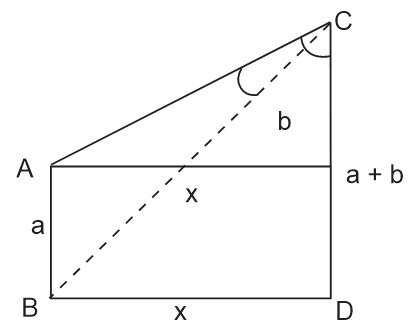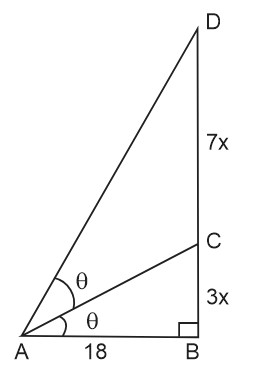Filter By

## All Questions

#### The value ofis : Option: 1Option: 2Option: 3Option: 4Trigonometric Identities -

Trigonometric Identities-

These identities are the equations that hold true regardless of the angle being chosen.-

Allied Angles (Part 1) -

Allied Angles (Part 1)

Two angles or numbers are called allied iff their sum or difference is a multiple of π/2

• sin (900 - θ) = cos (θ)

• cos (900 - θ) = sin (θ)

• tan (900 - θ) = cot (θ)

• csc (900 - θ) = sec (θ)

• sec (900 - θ) = csc (θ)

• cot (900 - θ) = tan (θ)

• sin (900 + θ) = cos (θ)

• cos (900 + θ) = - sin (θ)

• tan (900 + θ) = - cot (θ)

• csc (900 + θ) = sec (θ)

• sec (900 + θ) = - csc (θ)

• cot (900 + θ) = - tan (θ)

-#### Ifandthenis equal to ________. Option: 1Option: 2Option: 3Option: 4Trigonometric Identities -

Trigonometric Identities-

These identities are the equations that hold true regardless of the angle being chosen.-

Double Angle Formula and Reduction Formula -

Double Angle Formula and Reduction Formula-Correct Option (2)

## Crack CUET with india's "Best Teachers"

• HD Video Lectures
• Unlimited Mock Tests
• Faculty Support#### Two poles,of length a metres andof lengthmetres are erected at the same horizontal level with bases at. Ifand, then : Option: 1Option: 2Option: 3Option: 4## JEE Main high-scoring chapters and topics

Study 40% syllabus and score up to 100% marks in JEE

Using graphs of

## NEET 2024 Most scoring concepts

Just Study 32% of the NEET syllabus and Score up to 100% marks

#### The domain of the functionis :Option: 1Option: 2Option: 3Option: 4Domain

..........(1)

.......(2)

From (1) and (2)

## Crack CUET with india's "Best Teachers"

• HD Video Lectures
• Unlimited Mock Tests
• Faculty Support#### A vertical pole fixed to the horizontal ground is divided in the ratioby a mark on it with lower part shorter than the upper part. If the two parts subtend equal angles at a point on the groundaway from the base of the pole, then the height of the pole (in meters) is :Option: 1Option: 2Option: 3Option: 4option (3)

####is a root of the equation :Option: 1Option: 2Option: 3Option: 4option (3)